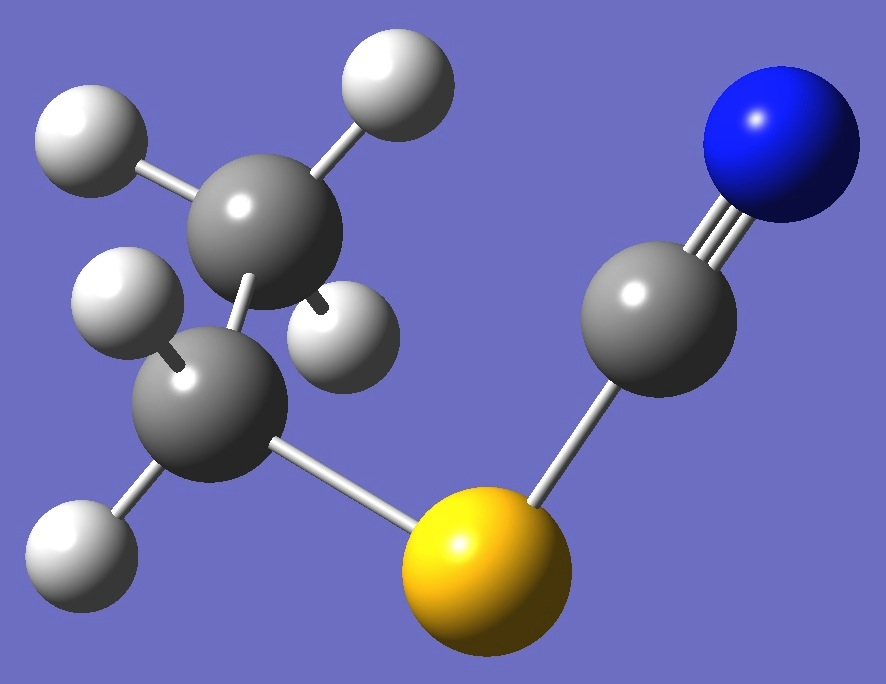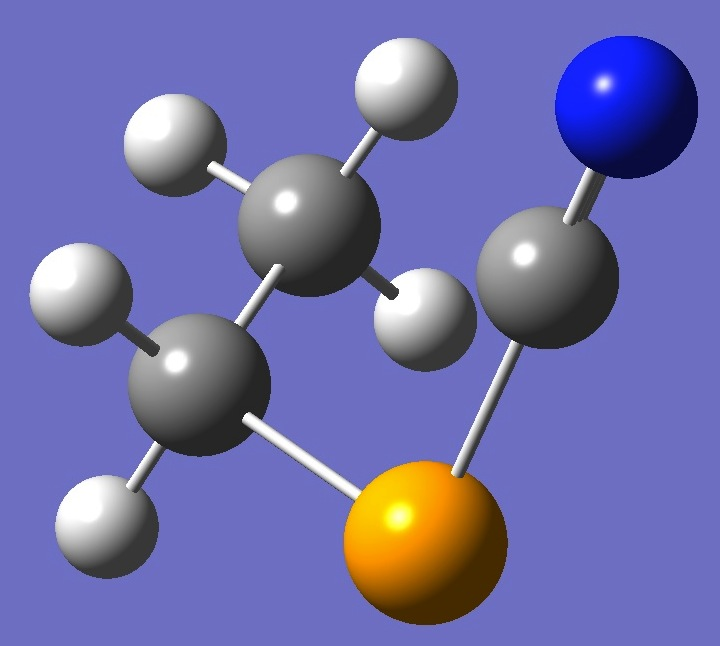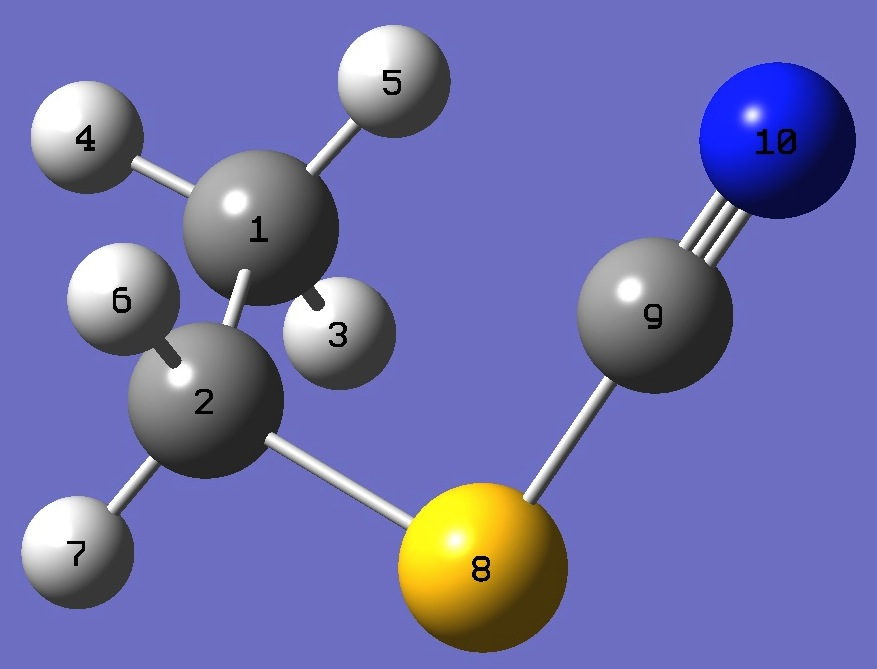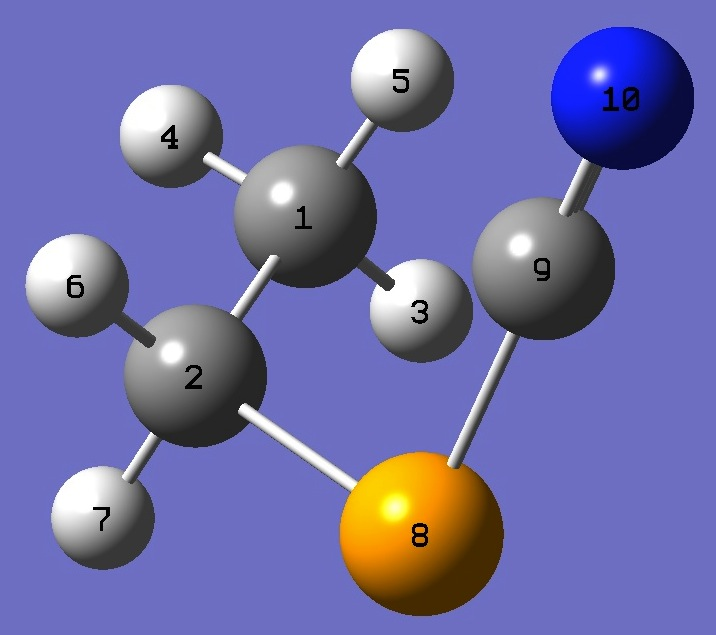CH3CH2-X-CN: X=S,Se

Nitrogen

Nuclear Quadrupole Coupling Constants

in Ethyl Thio- and Seleno-cyanate

The microwave spectrum of ethyl thiocyanate was observed and assigned by Bjørseth and Marstokk , and ethyl selenocyanate by Sakaizuma and Itakura .  Rotational constants and dipole moments were determined.

For each title molecule, shown below, calculation of the nitrogen nqcc tensor was made on a molecular structures given by MP2/6-311+G(3df,3pd) optimization with empirically corrected approximate equilibrium single CC and triple CN bond lengths.  These calculated nqcc's are given in Tables 1 and 2.  Structure parameters are given in Z-Matrix format in Table 3, rotational constants and dipole moments in Table 4.

Ethyl thiocyanate

Ethyl selenocyanateDihedral CCSC = 61.9o

Dihedral CCSeC = 60.6o

In Tables 1 and 2, subscripts a,b,c refer to the principal axes of the inertia tensor; x,y,z to the principal axes of the nqcc tensor.  ETA = (Xxx - Xyy)/Xzz.  Ø (degrees) is the angle between its subscripted parameters.

RSD is the calibration residual standard deviation of the B3PW91/6-311+G(df,pd) model for calculation of the nitrogen efg's/nqcc's.

 Table 1.  Nitrogen nqcc tensor in Ethyl thiocyanate (MHz).  Calculation was made on  molecular structure given by MP2/6-311+G(3df,3pd) optimization, with approximate re single CC and triple CN bond lengths. Calc Expt 14N Xaa - 0.661 Xbb - 0.603 Xcc 1.264 Xab - 3.322 Xac - 0.830 Xbc 0.176 RSD 0.030 (1.3 %) Xxx 1.008 Xyy 2.987 Xzz - 3.996 ETA 0.495 Øz,CN 0.40

 Table 2.  Nitrogen nqcc tensor in Ethyl selenocyanate (MHz).  Calculation was made on  molecular structure given by MP2/6-311+G(3df,3pd) optimization, with approximate re single CC and triple CN bond lengths. Calc Expt 14N Xaa - 0.459 Xbb - 0.924 Xcc 1.383 Xab - 3.414 Xac - 0.566 Xbc 0.147 RSD 0.030 (1.3 %) Xxx 1.223 Xyy 2.905 Xzz - 4.128 ETA 0.408 Øz,CN 0.31

 Table 3.  CH3CH2-X-CN, X=S,Se:  MP2/6-311+G(3df,3pd) optimized structure parameters (Å and degrees).  Approximate re bond lenths are given in parentheses. Ethyl thiocyanate Ethyl selenocyanateC            C,1,B1            H,1,B2,2,A1            H,1,B3,2,A2,3,D1,0            H,1,B4,2,A3,4,D2,0            H,2,B5,1,A4,5,D3,0            H,2,B6,1,A5,5,D4,0            X,2,B7,1,A6,5,D5,0            C,8,B8,2,A7,1,D6,0            N,9,B9,2,A8,1,D7,0B1=1.51599151     (1.5141)  B2=1.08763703  B3=1.08936661  B4=1.08841553  B5=1.08767378  B6=1.08993972  B7=1.82024887  B8=1.68386981  B9=1.17643598     (1.1609)  A1=110.87852519  A2=109.65244951  A3=110.69143711  A4=112.13954362  A5=111.66827931  A6=113.23780586  A7=98.09485669  A8=137.76991586  D1=120.10162914  D2=119.32171762  D3=60.38617404  D4=-177.89600381  D5=-62.07899607  D6=61.92291678  D7=53.80291966 B1=1.51563583     (1.5138)  B2=1.0877583  B3=1.08996879  B4=1.08848716  B5=1.08701077  B6=1.0899813  B7=1.96003924  B8=1.83238579  B9=1.17617212     (1.1607)  A1=111.05161745  A2=109.61248305  A3=110.82449669  A4=112.42612556  A5=112.04826818  A6=113.11508786  A7=94.96373213  A8=135.58561198  D1=120.04935107  D2=119.28079231  D3=59.85828092  D4=-177.62396892  D5=-61.65721097  D6=60.55745631  D7=52.64307017

 Table 4. CH3CH2-X-CN, X=S,Se: Rotational Constants (MHz) and Dipole Moments (D). ____CH3CH2-S-CN____ ____CH3CH2-Se-CN____ Calc Expt  Calc Expt  A 6259 6283.90(2) 3881 3922.92(18) B 3154 3083.540(9) 2978 2898.00(8) C 2281 2245.224(9) 1811 1789.82(2) |µa| 4.17 3.80(10) 4.21 4.37(10) |µb| 1.08 1.13(5) 1.41 0.80(5) |µc| 0.33 0.61(4) 0.30 0.50(5)

 A.Bjørseth and K.M.Marstokk, J.Mol.Struct. 11,15(1972).

 T.Sakaizuma and T.Itakura, J.Mol.Spectrosc. 163,1(1994).

Ethylcyanate
HSCN
ClSCN
BrSCN

Propargylthiocyanate Propargylselenocyanate

Table of Contents

Molecules/Nitrogen

CH3CH2XCN.html

Last Modified 17 Feb 2014